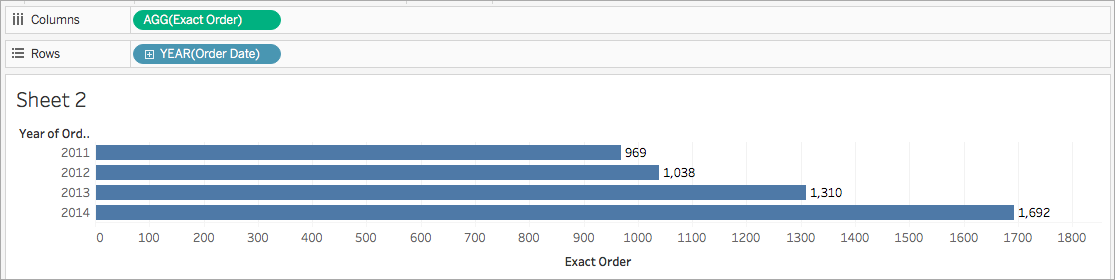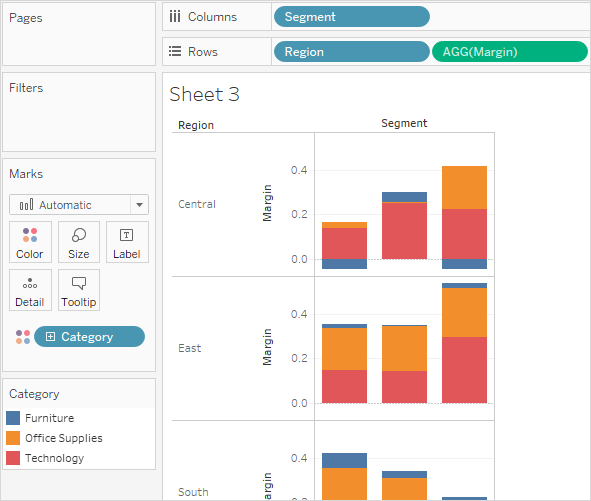# Aggregate Functions in Tableau

This article introduces aggregate functions and their uses in Tableau. It also demonstrates how to create an aggregate calculation using an example.

## Why use aggregate functions

Aggregate functions allow you to summarize or change the granularity of your data.

For example, you might want to know exactly how many orders your store had for a particular year. You can use the COUNTD function to summarize the exact number of orders your company had, and then break the visualization down by year.

The calculation might look something like this:

`COUNTD(Order ID)`

The visualization might look something like this:## Aggregate functions available in Tableau

Aggregations and floating-point arithmetic: The results of some aggregations may not always be exactly as expected. For example, you may find that the Sum function returns a value such as -1.42e-14 for a column of numbers that you know should sum to exactly 0. This happens because the Institute of Electrical and Electronics Engineers (IEEE) 754 floating-point standard requires that numbers be stored in binary format, which means that numbers are sometimes rounded at extremely fine levels of precision. You can eliminate this potential distraction by using the ROUND function (see Number Functions) or by formatting the number to show fewer decimal places.

#### Definition

ATTR

`ATTR(expression)`

Returns the value of the expression if it has a single value for all rows. Otherwise returns an asterisk. Null values are ignored.

AVG

`AVG(expression)`

Returns the average of all the values in the expression. AVG can be used with numeric fields only. Null values are ignored.

COLLECT

`COLLECT (spatial)`

An aggregate calculation that combines the values in the argument field. Null values are ignored.

Note: The COLLECT function can only be used with spatial fields.

Example:

`COLLECT ([Geometry])`

CORR

`CORR(expression 1, expression2)`

Returns the Pearson correlation coefficient of two expressions.

The Pearson correlation measures the linear relationship between two variables. Results range from -1 to +1 inclusive, where 1 denotes an exact positive linear relationship, as when a positive change in one variable implies a positive change of corresponding magnitude in the other, 0 denotes no linear relationship between the variance, and −1 is an exact negative relationship.

CORR is available with the following data sources:

• Tableau data extracts (you can create an extract from any data source)
• Cloudera Hive
• EXASolution
• Firebird (version 3.0 and later)
• IBM PDA (Netezza)
• Oracle
• PostgreSQL
• Presto
• SybaseIQ
• Vertica

For other data sources, consider either extracting the data or using WINDOW_CORR. See Table Calculation Functions.

Note: The square of a CORR result is equivalent to the R-Squared value for a linear trend line model. See Trend Line Model Terms.

Example:

You can use CORR to visualize correlation in a disaggregated scatter plot. The way to do this is to use a table-scoped level of detail expression. For example:

`{CORR(Sales, Profit)}`

With a level of detail expression, the correlation is run over all rows. If you used a formula like `CORR(Sales, Profit)` (without the surrounding brackets to make it a level of detail expression), the view would show the correlation of each individual point in the scatter plot with each other point, which is undefined.

See Table-Scoped

COUNT

`COUNT(expression)`

Returns the number of items in a group. Null values are not counted.

COUNTD

`COUNTD(expression)`

Returns the number of distinct items in a group. Null values are not counted. This function is not available in the following cases: workbooks created before Tableau Desktop 8.2 that use Microsoft Excel or text file data sources, workbooks that use the legacy connection, and workbooks that use Microsoft Access data sources. Extract your data into an extract file to use this function. See Extract Your Data.

COVAR

`COVAR(expression 1, expression2)`

Returns the sample covariance of two expressions.

Covariance quantifies how two variables change together. A positive covariance indicates that the variables tend to move in the same direction, as when larger values of one variable tend to correspond to larger values of the other variable, on average. Sample covariance uses the number of non-null data points n - 1 to normalize the covariance calculation, rather than n, which is used by the population covariance (available with the COVARP function). Sample covariance is the appropriate choice when the data is a random sample that is being used to estimate the covariance for a larger population.

COVAR is available with the following data sources:

• Tableau data extracts (you can create an extract from any data source)
• Cloudera Hive
• EXASolution
• Firebird (version 3.0 and later)
• IBM PDA (Netezza)
• Oracle
• PostgreSQL
• Presto
• SybaseIQ
• Vertica

For other data sources, consider either extracting the data or using WINDOW_COVAR. See Table Calculation Functions.

If expression1 and expression2 are the same—for example, COVAR([profit], [profit])—COVAR returns a value that indicates how widely values are distributed.

Note: The value of COVAR(X, X) is equivalent to the value of VAR(X) and also to the value of STDEV(X)^2.

Example:

The following formula returns the sample covariance of Sales and Profit.

`COVAR([Sales], [Profit])`

COVARP

`COVARP(expression 1, expression2)`

Returns the population covariance of two expressions.

Covariance quantifies how two variables change together. A positive covariance indicates that the variables tend to move in the same direction, as when larger values of one variable tend to correspond to larger values of the other variable, on average. Population covariance is sample covariance multiplied by (n-1)/n, where n is the total number of non-null data points. Population covariance is the appropriate choice when there is data available for all items of interest as opposed to when there is only a random subset of items, in which case sample covariance (with the COVAR function) is appropriate.

COVARP is available with the following data sources:

• Tableau data extracts (you can create an extract from any data source)
• Cloudera Hive
• EXASolution
• Firebird (version 3.0 and later)
• IBM PDA (Netezza)
• Oracle
• PostgreSQL
• Presto
• SybaseIQ
• Vertica

For other data sources, consider either extracting the data or using WINDOW_COVARP. See Table Calculation Functions.

If expression1 and expression2 are the same—for example, COVARP([profit], [profit])—COVARP returns a value that indicates how widely values are distributed.

Note: The value of COVARP(X, X) is equivalent to the value of VARP(X) and also to the value of STDEVP(X)^2.

Example:

The following formula returns the population covariance of Sales and Profit.

`COVARP([Sales], [Profit])`

MAX

`MAX(expression)`

Returns the maximum of an expression across all records. If the expression is a string value, this function returns the last value where last is defined by alphabetical order.

MEDIAN

`MEDIAN(expression)`

Returns the median of an expression across all records. Median can only be used with numeric fields. Null values are ignored. This function is not available for workbooks created before Tableau Desktop 8.2 or that use legacy connections. It is also not available for connections using any of the following data sources:

• Access
• Amazon Redshift
• HP Vertica
• IBM DB2
• IBM PDA (Netezza)
• Microsoft SQL Server
• MySQL
• SAP HANA

For other data source types, you can extract your data into an extract file to use this function. See Extract Your Data.

MIN

`MIN(expression)`

Returns the minimum of an expression across all records. If the expression is a string value, this function returns the first value where first is defined by alphabetical order.

PERCENTILE

`PERCENTILE(expression, number)`

Returns the percentile value from the given expression corresponding to the specified number. The number must be between 0 and 1 (inclusive)—for example, 0.66, and must be a numeric constant.

This function is available for the following data sources.

• Non-legacy Microsoft Excel and Text File connections.

• Extracts and extract-only data source types (for example, Google Analytics, OData, or Salesforce).

• Sybase IQ 15.1 and later data sources.

• Oracle 10 and later data sources.

• Cloudera Hive and Hortonworks Hadoop Hive data sources.

• EXASolution 4.2 and later data sources.

For other data source types, you can extract your data into an extract file to use this function. See Extract Your Data.

STDEV

`STDEV(expression)`

Returns the statistical standard deviation of all values in the given expression based on a sample of the population.

STDEVP

`STDEVP(expression)`

Returns the statistical standard deviation of all values in the given expression based on a biased population.

SUM

`SUM(expression)`

Returns the sum of all values in the expression. SUM can be used with numeric fields only. Null values are ignored.

VAR

`VAR(expression)`

Returns the statistical variance of all values in the given expression based on a sample of the population.

VARP

`VARP(expression)`

Returns the statistical variance of all values in the given expression on the entire population.

## Create an aggregate calculation

Follow along with the steps below to learn how to create an aggregate calculation.

1. In Tableau Desktop, connect to the Sample - Superstore saved data source, which comes with Tableau.

2. Navigate to a worksheet and select Analysis > Create Calculated Field.

3. In the calculation editor that opens, do the following:

• Name the calculated field Margin.

• Enter the following formula:

```IIF(SUM([Sales]) !=0, SUM([Profit])/SUM([Sales]), 0)```

Note: You can use the function reference to find and add aggregate functions and other functions (like the logical IIF function in this example) to the calculation formula. For more information, see Use the functions reference in the calculation editor.

• When finished, click OK.

The new aggregate calculation appears under Measures in the Data pane. Just like your other fields, you can use it in one or more visualizations.

Note: Aggregation calculations are always measures.

When Margin is placed on a shelf or card in the worksheet, its name is changed to AGG(Margin), which indicates that it is an aggregate calculation and cannot be aggregated any further.## Rules for aggregate calculations

The rules that apply to aggregate calculations are as follows:

• For any aggregate calculation, you cannot combine an aggregated value and a disaggregated value. For example, SUM(Price)*[Items] is not a valid expression because SUM(Price) is aggregated and Items is not. However, SUM(Price*Items) and SUM(Price)*SUM(Items) are both valid.

• Constant terms in an expression act as aggregated or disaggregated values as appropriate. For example: SUM(Price*7) and SUM(Price)*7 are both valid expressions.

• All of the functions can be evaluated on aggregated values. However, the arguments to any given function must either all be aggregated or all disaggregated. For example: MAX(SUM(Sales),Profit) is not a valid expression because Sales is aggregated and Profit is not. However, MAX(SUM(Sales),SUM(Profit)) is a valid expression.

• The result of an aggregate calculation is always a measure.

• Like predefined aggregations, aggregate calculations are computed correctly for grand totals. Refer to Grand Totals for more information.